• 卷积网络的提出： 《Adaptive deconvolutional networks for mid and high level feature learning》 1. 该文处理的问题 a. 问题： 最近两年深层的卷积神经网络，进展非常惊人，在计算机视觉...
原文地址：地址1，本文在此基础上进行了整理和划重点

本文文献：Zeiler, Matthew D.; Fergus, Rob,"Visualizing and Understanding Convolutional Networks".  In: Proc. European Conference on Computer Vision (ECCV), pp. 1-14, 2014. Zurich, Switzerland.

反卷积网络的提出：《Adaptive deconvolutional networks for mid and high level feature learning》

1. 该文处理的问题

a. 问题：

最近两年深层的卷积神经网络，进展非常惊人，在计算机视觉方面，识别精度不断的突破，CVPR上的关于CNN的文献一大堆。比如调整了某个参数，CNN结果精度飙升，但如果别人问你，为什么这样调参精度会飙升呢，你所设计的CNN到底学习到了什么特征？

b. 处理：

这篇文献的目的，就是要通过特征可视化，查看你的精度确实提高了，看到你设计的CNN学习到的特征。

2. 该文采取的思路和方法，及为什么能解决所处理的问题？

a. 采取的思路和方法

前提：

反卷积网络在文献是用于无监督学习的。然而本文的反卷积过程并不具备学习的能力，仅仅是用于可视化一个已经训练好的卷积网络模型，没有学习训练的过程。

过程总述：

输入（各层得到的特征图）——> 过程（反池化，反激活，反卷积）——> 输出（反卷积结果，可视化各层得到的特征图）

过程具体：

1. 反池化

池化本质是不可逆的过程，在这里采用近似方法模拟可逆的过程。首先记录池化过程中，最大激活值得坐标位置。然后在反池化的时候，只把池化过程中最大激活值所在的位置坐标的值激活，其它的像素值置为0。因为在池化的过程中，除了最大值所在的位置，其它的值也是不为0的，所以上述操作只是一种近似。示意图：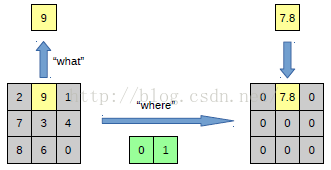以上面的图片为例，上面的图片中左边表示pooling过程，右边表示unpooling过程。

假设我们pooling块的大小是3*3，采用max pooling后，可以得到一个输出神经元其激活值为9，pooling是一个下采样的过程，本来是3*3大小，经过pooling后，就变成了1*1大小的图片了。

upooling刚好与pooling过程相反，它是一个上采样的过程，是pooling的一个反向运算，当我们由一个神经元要扩展到3*3个神经元的时候，我们需要借助于pooling过程中，记录下的最大值所在的位置坐标(0,1)，然后在unpooling过程的时候，就把(0,1)这个像素点的位置填上去，其它的神经元激活值全部为0。

再来一个例子：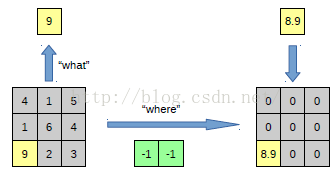在max pooling的时候，我们不仅要得到最大值，同时还要记录下最大值的坐标 (-1-1)，然后在unpooling的时候，就直接把(-1-1) 这个点的值填到对应位置，其它的激活值全部为0。

2. 反激活

我们在Alexnet中，relu函数是用于保证每层输出的激活值都是正数，因此对于反向过程，我们同样需要保证每层的特征图为正值，也就是说这个反激活过程和激活过程没有什么差别，都是直接采用relu函数。

3. 反卷积

反卷积过程的滤波器，采用卷积过程的滤波器的转置(参数一样，只不过把参数矩阵水平和垂直方向翻转了)

网络的整个过程：

输入图片 —> 卷积 —> Relu —> 最大池化 —>

|

图片<— 反卷积 <— Relu <— 反池化 <— 特征图

b. 为什么能解决所处理的问题

已有方法的特征：

一类方法集中于研究训练反卷及网络的图像语义分割(将像素还原成图像)
一类方法集中于研究训练反卷积网络从而生成图像或者图形
本文方法的创新之处：

然而本文是用于可视化一个已经训练好的卷积网络模型 (展示每一层的特征图)，没有学习训练的过程。


展开全文• 卷积神经网络的各层卷积单元在模型网络中实际上有充当了目标检测器的作用，尽管没有提供对目标位置的监督。虽然其拥有在卷积层中定位对象的非凡能力，但当使用全连接层进行分类时，这种能力就会丧失。基于此，提出了...

本文首发于：行者AI

在整篇文章论述开始之前，我们先做一些概念性的讲解铺垫。卷积神经网络的各层卷积单元在模型网络中实际上有充当了目标检测器的作用，尽管没有提供对目标位置的监督。虽然其拥有在卷积层中定位对象的非凡能力，但当使用全连接层进行分类时，这种能力就会丧失。基于此，提出了CAM（类激活映射）的概念，采用全局平均池化，以热力图的形式告诉我们，模型通过哪些像素点得知图片属于某个类别，使模型透明化和具有可解释性，如下图所示：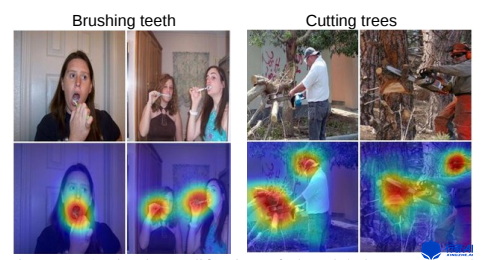1. Global Average Pooling的工作机制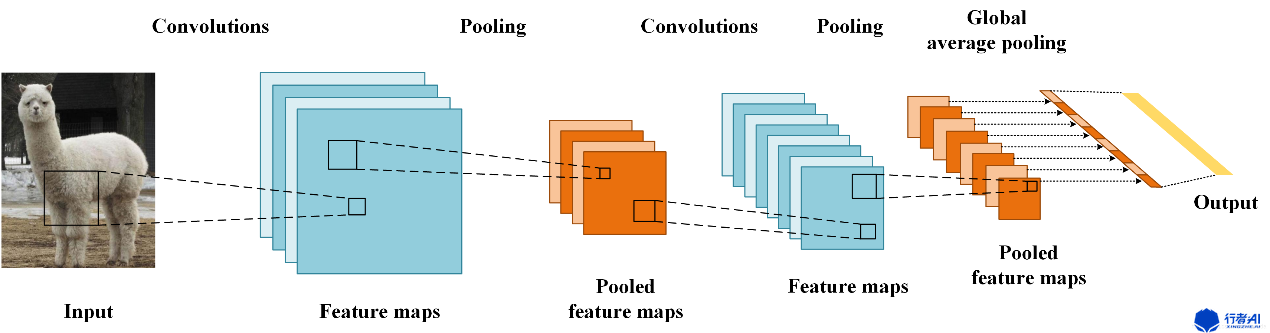这里我们假设最后的类别数为n，最后一层含有n个特征图，求每张特征图所有像素的平均值，后接入一个有n个神经元的全连接层。要有n个特征图的原因在于，每个特征图主要提取了某一类别相关的某些特征。
2. 什么是CAM？
CNN最后一层特征图富含有最为丰富类别语意信息（可以理解为高度抽象的类别特征），因此CAM基于最后一层特征图进行可视化。CAM能让我们对CNN网络有很好的解释作用，利用特征图权重叠加的原理获得热图，详细工作原理如下图所示。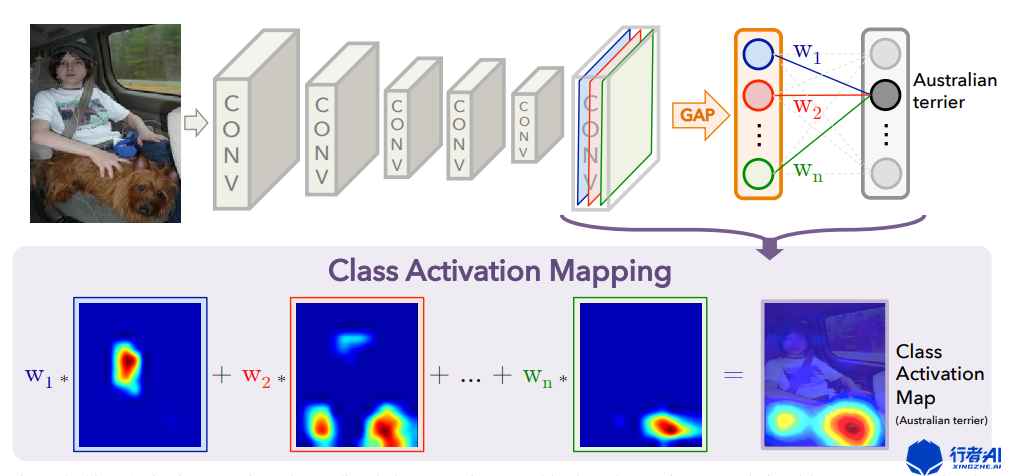设最后一层有n张特征图，记为$A^1,A^2,...A^n$，分类层中一个神经元有n个权重，一个神经元对应一类，设第$i$个神经元的权重为$w^1，w^2,...w^n$，则第c类的CAM的生成方式为：
$L_{CAM}^c = \sum_{i=1}^n w_i^cA^i (式1)$
生成的CAM大小与最后一层特征图的大小一致，接着进行上采样即可得到与原图大小一致的CAM。
2.1. 为什么如此计算可以得到类别相关区域
用GAP表示全局平均池化函数，沿用上述符号，第c类的分类得分为$S_c$，GAP的权重为$w_i^c$，特征图大小为$c_1*c_2$，第$i$个特征图第$k$行第$j$列的像素值为$A_{kj}^i$，则有：
$S_c = \sum_{i=1}^n w_i^c GAP(A_i)$
$= \sum_{i=1}^n w_i^c \frac 1Z \sum_{k=1}^{c_1} \sum_{j=1}^{c_2}A_{kj}^i$
$= \frac 1Z \sum_{i=1}^n \sum_{k=1}^{c_1} \sum_{j=1}^{c_2} w_i^c A_{kj}^i (式2)$
特征图中的一个像素对应原图中的一个区域，而像素值表示该区域提取到的特征，由上式可知$S_c$的大小由特征图中像素值与权重决定，特征图中像素值与权重的乘积大于0，有利于将样本分到该类，即CNN认为原图中的该区域具有类别相关特征。式1就是计算特征图中的每个像素值是否具有类别相关特征，如果有，我们可以通过上采样，看看这个像素对应的是原图中的哪一部分。GAP的出发点也是如此，即在训练过程中让网络学会判断原图中哪个区域具有类别相关特征，由于GAP去除了多余的全连接层，并且没有引入参数，因此GAP可以降低过拟合的风险。可视化的结果也表明，CNN正确分类的确是因为注意到了原图中正确的类别相关特征。
2.2. CAM缺陷
需要修改网络结构并重新训练模型，导致在实际应用中并不方便。
3. Grad-CAM
3.1. Grad-CAM结构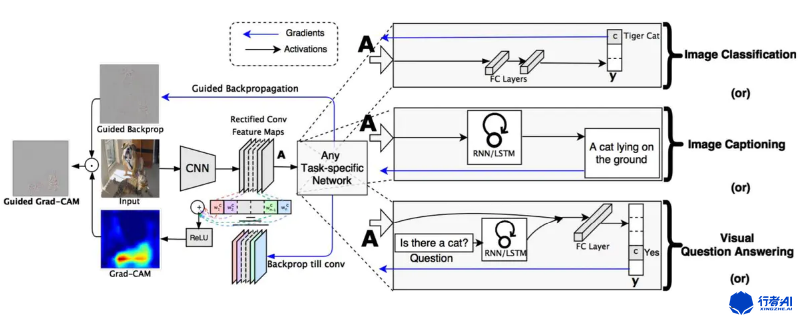Grad-CAM 和 CAM 基本思路一样，区别就在于如何获取每个特征图的权重，采用了梯度的全局平均来计算权重。定义了Grad-CAM中第$\kappa$个特征图对应类别c的权重：
$\alpha_\kappa^c = \frac 1Z \sum_i \sum_j \frac {y^c}{\alpha A_{ij}^k} (式3)$
其中，Z表示特征图像素个数，$y^c$表示第c类得分梯度，$A_{ij}^k$表示第$k$个特征图中，$(i,j)$位置处的像素值。然后再求得所有的特征图对应的类别的权重后进行加权求和，这样便可以得到最后的热力图，求和公式如下：
$L_{Grad-CAM}^c = ReLU(\sum_k \alpha_k^c A^k)(式4)$
3.2. Grad-CAM效果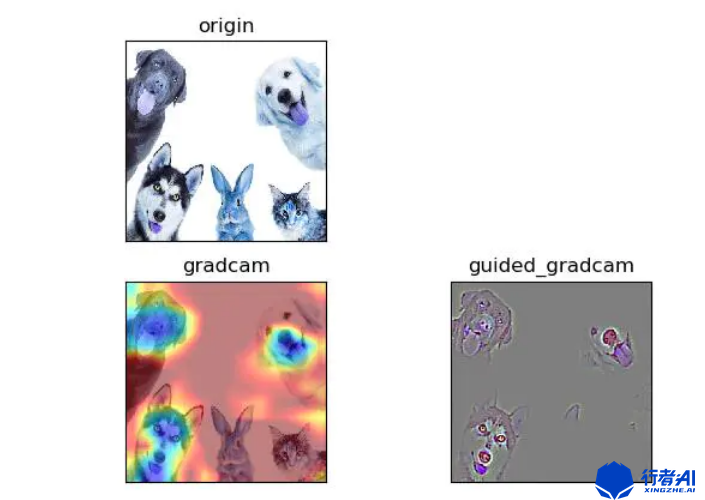4. 结论
可视化可以进一步区别分类，准确地更好地揭示分类器的可信赖性，并帮助识别数据集中的偏差。真正的AI应用，也更应该让人们信任和使用它的行为。
5. 代码实现
https://github.com/jacobgil/keras-cam
参考文献

B.     Zhou, A. Khosla, A. Lapedriza, A. Oliva, and A. Torralba. Object detectors     emerge in deep scene cnns. International Conference on Learning     Representations, 2015.

Computers     - Computer Graphics; Investigators from Georgia Institute of Technology     Have Reported New Data on Computer Graphics (Grad-CAM: Visual Explanations     from Deep Networks via Gradient-Based Localization). 2020, :355-.

PS：更多技术干货，快关注【公众号 | xingzhe_ai】，与行者一起讨论吧！


展开全文深度学习
• 本文主要是实现了一个简单的卷积神经网络,并对卷积过程中提取特征进行了可视化. 卷积神经网络最早是为了解决图像识别问题,现在也用在时间序列数据和文本数据处理当中,卷积神经网络对于数据特征提取不用额外...
版权声明：本文为博主原创文章，未经博主允许不得转载。
本文主要是实现了一个简单的卷积神经网络,并对卷积过程中的提取特征进行了可视化.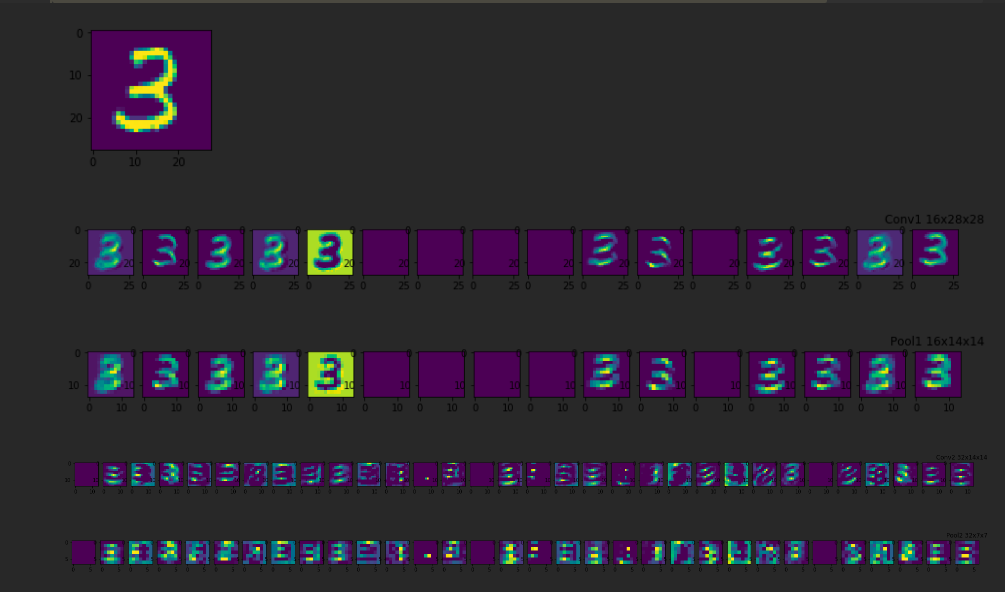卷积神经网络最早是为了解决图像识别的问题,现在也用在时间序列数据和文本数据处理当中,卷积神经网络对于数据特征的提取不用额外进行,在对网络的训练的过程当中,网络会自动提取主要的特征.
卷积神经网络直接用原始图像的全部像素作为输入,但是内部为非全连接结构.因为图像数据在空间上是有组织结构的,每一个像素在空间上和周围的像素是有关系的,和相距很远的像素基本上是没什么联系的,每个神经元只需要接受局部的像素作为输入,再将局部信息汇总就能得到全局信息.
权值共享和池化两个操作使网络模型的参数大幅的减少,提高了模型的训练效率.
权值共享:
在卷积层中可以有多个卷积核,每个卷积核与原始图像进行卷积运算后会映射出一个新的2D图像,新图像的每个像素都来自同一个卷积核.这就是权值共享.
池化:
降采样,对卷积(滤波)后,经过激活函数处理后的图像,保留像素块中灰度值最高的像素点(保留最主要的特征),比如进行 2X2的最大池化,把一个2x2的像素块降为1x1的像素块.
# 卷积网络的训练数据为MNIST(28*28灰度单色图像)
import tensorflow as tf
import numpy as np
import matplotlib.pyplot as plt
from tensorflow.examples.tutorials.mnist import input_data


训练参数
train_epochs = 100    # 训练轮数
batch_size   = 100     # 随机出去数据大小
display_step = 1       # 显示训练结果的间隔
learning_rate= 0.0001  # 学习效率
drop_prob    = 0.5     # 正则化,丢弃比例
fch_nodes    = 512     # 全连接隐藏层神经元的个数

网络结构
[外链图片转存失败,源站可能有防盗链机制,建议将图片保存下来直接上传(img-GPwo1L58-1579072744365)(output_16_11.png)]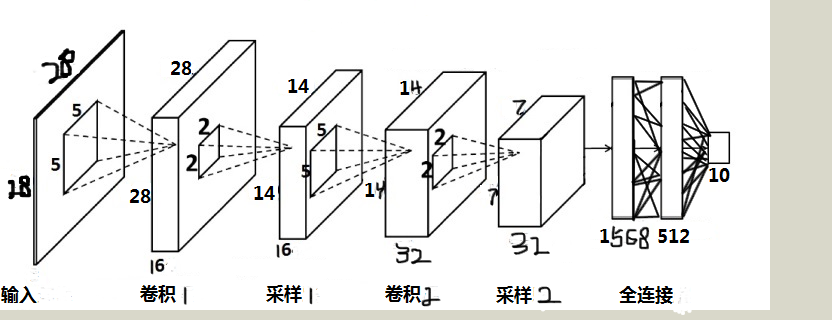输入层为输入的灰度图像尺寸:  -1 x 28 x 28 x 1
第一个卷积层,卷积核的大小,深度和数量 (5, 5, 1, 16)
池化后的特征张量尺寸:       -1 x 14 x 14 x 16
第二个卷积层,卷积核的大小,深度和数量 (5, 5, 16, 32)
池化后的特征张量尺寸:       -1 x 7 x 7 x 32
全连接层权重矩阵         1568 x 512
输出层与全连接隐藏层之间,  512 x 10

一些辅助函数
# 网络模型需要的一些辅助函数
# 权重初始化(卷积核初始化)
# tf.truncated_normal()不同于tf.random_normal(),返回的值中不会偏离均值两倍的标准差
# 参数shpae为一个列表对象,例如[5, 5, 1, 32]对应
# 5,5 表示卷积核的大小, 1代表通道channel,对彩色图片做卷积是3,单色灰度为1
# 最后一个数字32,卷积核的个数,(也就是卷基层提取的特征数量)
#   显式声明数据类型,切记
def weight_init(shape):
weights = tf.truncated_normal(shape, stddev=0.1,dtype=tf.float32)
return tf.Variable(weights)

# 偏置的初始化
def biases_init(shape):
biases = tf.random_normal(shape,dtype=tf.float32)
return tf.Variable(biases)

# 随机选取mini_batch
def get_random_batchdata(n_samples, batchsize):
start_index = np.random.randint(0, n_samples - batchsize)
return (start_index, start_index + batchsize)

# 全连接层权重初始化函数xavier
def xavier_init(layer1, layer2, constant = 1):
Min = -constant * np.sqrt(6.0 / (layer1 + layer2))
Max = constant * np.sqrt(6.0 / (layer1 + layer2))
return tf.Variable(tf.random_uniform((layer1, layer2), minval = Min, maxval = Max, dtype = tf.float32))

# 卷积
def conv2d(x, w):
return tf.nn.conv2d(x, w, strides=[1, 1, 1, 1], padding='SAME')

# 源码的位置在tensorflow/python/ops下nn_impl.py和nn_ops.py
# 这个函数接收两个参数,x 是图像的像素, w 是卷积核
# x 张量的维度[batch, height, width, channels]
# w 卷积核的维度[height, width, channels, channels_multiplier]
# tf.nn.conv2d()是一个二维卷积函数,
# stirdes 是卷积核移动的步长,4个1表示,在x张量维度的四个参数上移动步长
# padding 参数'SAME',表示对原始输入像素进行填充,卷积后映射的2D图像与原图大小相等
# 填充,是指在原图像素值矩阵周围填充0像素点
# 如果不进行填充,假设 原图为 32x32 的图像,卷积和大小为 5x5 ,卷积后映射图像大小 为 28x28

Padding
卷积核在提取特征时的动作成为padding，它有两种方式：SAME和VALID。卷积核的移动步长不一定能够整除图片像素的宽度，所以在有些图片的边框位置有些像素不能被卷积。这种不越过边缘的取样就叫做 valid padding，卷积后的图像面积小于原图像。为了让卷积核覆盖到所有的像素，可以对边缘位置进行0像素填充，然后在进行卷积。这种越过边缘的取样是 same padding。如过移动步长为1，那么得到和原图一样大小的图像。
如果步长很大，超过了卷积核长度，那么same padding，得到的特征图也会小于原来的图像。

# 池化
def max_pool_2x2(x):
return tf.nn.max_pool(x, ksize=[1, 2, 2, 1], strides=[1, 2, 2, 1], padding='SAME')

# 池化跟卷积的情况有点类似
# x 是卷积后,有经过非线性激活后的图像,
# ksize 是池化滑动张量
# ksize 的维度[batch, height, width, channels],跟 x 张量相同
# strides [1, 2, 2, 1],与上面对应维度的移动步长
# padding与卷积函数相同,padding='VALID',对原图像不进行0填充

# x 是手写图像的像素值,y 是图像对应的标签
x = tf.placeholder(tf.float32, [None, 784])
y = tf.placeholder(tf.float32, [None, 10])
# 把灰度图像一维向量,转换为28x28二维结构
x_image = tf.reshape(x, [-1, 28, 28, 1])
# -1表示任意数量的样本数,大小为28x28深度为一的张量
# 可以忽略(其实是用深度为28的,28x1的张量,来表示28x28深度为1的张量)

第一层卷积+池化

w_conv1 = weight_init([5, 5, 1, 16])                             # 5x5,深度为1,16个
b_conv1 = biases_init()
h_conv1 = tf.nn.relu(conv2d(x_image, w_conv1) + b_conv1)    # 输出张量的尺寸:28x28x16
h_pool1 = max_pool_2x2(h_conv1)                                   # 池化后张量尺寸:14x14x16
# h_pool1 , 14x14的16个特征图

第二层卷积+池化

w_conv2 = weight_init([5, 5, 16, 32])                             # 5x5,深度为16,32个
b_conv2 = biases_init()
h_conv2 = tf.nn.relu(conv2d(h_pool1, w_conv2) + b_conv2)    # 输出张量的尺寸:14x14x32
h_pool2 = max_pool_2x2(h_conv2)                                   # 池化后张量尺寸:7x7x32
# h_pool2 , 7x7的32个特征图

全连接层
# h_pool2是一个7x7x32的tensor,将其转换为一个一维的向量
h_fpool2 = tf.reshape(h_pool2, [-1, 7*7*32])
# 全连接层,隐藏层节点为512个
# 权重初始化
w_fc1 = xavier_init(7*7*32, fch_nodes)
b_fc1 = biases_init([fch_nodes])
h_fc1 = tf.nn.relu(tf.matmul(h_fpool2, w_fc1) + b_fc1)

# 全连接隐藏层/输出层
# 为了防止网络出现过拟合的情况,对全连接隐藏层进行 Dropout(正则化)处理,在训练过程中随机的丢弃部分
# 节点的数据来防止过拟合.Dropout同把节点数据设置为0来丢弃一些特征值,仅在训练过程中,
# 预测的时候,仍使用全数据特征
# 传入丢弃节点数据的比例
#keep_prob = tf.placeholder(tf.float32)
h_fc1_drop = tf.nn.dropout(h_fc1, keep_prob=drop_prob)

# 隐藏层与输出层权重初始化
w_fc2 = xavier_init(fch_nodes, 10)
b_fc2 = biases_init()

# 未激活的输出
y_ = tf.add(tf.matmul(h_fc1_drop, w_fc2), b_fc2)
# 激活后的输出
y_out = tf.nn.softmax(y_)

# 交叉熵代价函数
cross_entropy = tf.reduce_mean(-tf.reduce_sum(y * tf.log(y_out), reduction_indices = ))

# tensorflow自带一个计算交叉熵的方法
# 输入没有进行非线性激活的输出值 和 对应真实标签
#cross_loss = tf.reduce_mean(tf.nn.softmax_cross_entropy_with_logits(y_, y))

# 优化器选择Adam(有多个选择)
optimizer = tf.train.AdamOptimizer(learning_rate).minimize(cross_entropy)

# 准确率
# 每个样本的预测结果是一个(1,10)的vector
correct_prediction = tf.equal(tf.argmax(y, 1), tf.argmax(y_out, 1))
# tf.cast把bool值转换为浮点数
accuracy = tf.reduce_mean(tf.cast(correct_prediction, tf.float32))

# 全局变量进行初始化的Operation
init = tf.global_variables_initializer()

# 加载数据集MNIST
mnist = input_data.read_data_sets('MNIST/mnist', one_hot=True)
n_samples = int(mnist.train.num_examples)
total_batches = int(n_samples / batch_size)

# 会话
with tf.Session() as sess:
sess.run(init)
Cost = []
Accuracy = []
for i in range(train_epochs):

for j in range(100):
start_index, end_index = get_random_batchdata(n_samples, batch_size)

batch_x = mnist.train.images[start_index: end_index]
batch_y = mnist.train.labels[start_index: end_index]
_, cost, accu = sess.run([ optimizer, cross_entropy,accuracy], feed_dict={x:batch_x, y:batch_y})
Cost.append(cost)
Accuracy.append(accu)
if i % display_step ==0:
print ('Epoch : %d ,  Cost : %.7f'%(i+1, cost))
print 'training finished'
# 代价函数曲线
fig1,ax1 = plt.subplots(figsize=(10,7))
plt.plot(Cost)
ax1.set_xlabel('Epochs')
ax1.set_ylabel('Cost')
plt.title('Cross Loss')
plt.grid()
plt.show()
# 准确率曲线
fig7,ax7 = plt.subplots(figsize=(10,7))
plt.plot(Accuracy)
ax7.set_xlabel('Epochs')
ax7.set_ylabel('Accuracy Rate')
plt.title('Train Accuracy Rate')
plt.grid()
plt.show()
#----------------------------------各个层特征可视化-------------------------------
# imput image
fig2,ax2 = plt.subplots(figsize=(2,2))
ax2.imshow(np.reshape(mnist.train.images, (28, 28)))
plt.show()

# 第一层的卷积输出的特征图
input_image = mnist.train.images[11:12]
conv1_16 = sess.run(h_conv1, feed_dict={x:input_image})     # [1, 28, 28 ,16]
conv1_transpose = sess.run(tf.transpose(conv1_16, [3, 0, 1, 2]))
fig3,ax3 = plt.subplots(nrows=1, ncols=16, figsize = (16,1))
for i in range(16):
ax3[i].imshow(conv1_transpose[i])                      # tensor的切片[row, column]

plt.title('Conv1 16x28x28')
plt.show()

# 第一层池化后的特征图
pool1_16 = sess.run(h_pool1, feed_dict={x:input_image})     # [1, 14, 14, 16]
pool1_transpose = sess.run(tf.transpose(pool1_16, [3, 0, 1, 2]))
fig4,ax4 = plt.subplots(nrows=1, ncols=16, figsize=(16,1))
for i in range(16):
ax4[i].imshow(pool1_transpose[i])

plt.title('Pool1 16x14x14')
plt.show()

# 第二层卷积输出特征图
conv2_32 = sess.run(h_conv2, feed_dict={x:input_image})          # [1, 14, 14, 32]
conv2_transpose = sess.run(tf.transpose(conv2_32, [3, 0, 1, 2]))
fig5,ax5 = plt.subplots(nrows=1, ncols=32, figsize = (32, 1))
for i in range(32):
ax5[i].imshow(conv2_transpose[i])
plt.title('Conv2 32x14x14')
plt.show()

# 第二层池化后的特征图
pool2_32 = sess.run(h_pool2, feed_dict={x:input_image})         #[1, 7, 7, 32]
pool2_transpose = sess.run(tf.transpose(pool2_32, [3, 0, 1, 2]))
fig6,ax6 = plt.subplots(nrows=1, ncols=32, figsize = (32, 1))
plt.title('Pool2 32x7x7')
for i in range(32):
ax6[i].imshow(pool2_transpose[i])

plt.show()


Epoch : 1 ,  Cost : 1.7629557
Epoch : 2 ,  Cost : 0.8955871
Epoch : 3 ,  Cost : 0.6002768
Epoch : 4 ,  Cost : 0.4222347
Epoch : 5 ,  Cost : 0.4106165
Epoch : 6 ,  Cost : 0.5070749
Epoch : 7 ,  Cost : 0.5032627
Epoch : 8 ,  Cost : 0.3399751
Epoch : 9 ,  Cost : 0.1524799
Epoch : 10 ,  Cost : 0.2328545
Epoch : 11 ,  Cost : 0.1815660
Epoch : 12 ,  Cost : 0.2749544
Epoch : 13 ,  Cost : 0.2539429
Epoch : 14 ,  Cost : 0.1850740
Epoch : 15 ,  Cost : 0.3227096
Epoch : 16 ,  Cost : 0.0711472
Epoch : 17 ,  Cost : 0.1688010
Epoch : 18 ,  Cost : 0.1442217
Epoch : 19 ,  Cost : 0.2415594
Epoch : 20 ,  Cost : 0.0848383
Epoch : 21 ,  Cost : 0.1879225
Epoch : 22 ,  Cost : 0.1355369
Epoch : 23 ,  Cost : 0.1578972
Epoch : 24 ,  Cost : 0.1017473
Epoch : 25 ,  Cost : 0.2265745
Epoch : 26 ,  Cost : 0.2625684
Epoch : 27 ,  Cost : 0.1950202
Epoch : 28 ,  Cost : 0.0607868
Epoch : 29 ,  Cost : 0.0782418
Epoch : 30 ,  Cost : 0.0744723
Epoch : 31 ,  Cost : 0.0848689
Epoch : 32 ,  Cost : 0.1038134
Epoch : 33 ,  Cost : 0.0848786
Epoch : 34 ,  Cost : 0.1219746
Epoch : 35 ,  Cost : 0.0889094
Epoch : 36 ,  Cost : 0.0605406
Epoch : 37 ,  Cost : 0.0478896
Epoch : 38 ,  Cost : 0.1100840
Epoch : 39 ,  Cost : 0.0168766
Epoch : 40 ,  Cost : 0.0479708
Epoch : 41 ,  Cost : 0.1187883
Epoch : 42 ,  Cost : 0.0707371
Epoch : 43 ,  Cost : 0.0471128
Epoch : 44 ,  Cost : 0.1206998
Epoch : 45 ,  Cost : 0.0674985
Epoch : 46 ,  Cost : 0.1218394
Epoch : 47 ,  Cost : 0.0840694
Epoch : 48 ,  Cost : 0.0468497
Epoch : 49 ,  Cost : 0.0899443
Epoch : 50 ,  Cost : 0.0111846
Epoch : 51 ,  Cost : 0.0653627
Epoch : 52 ,  Cost : 0.1446207
Epoch : 53 ,  Cost : 0.0320902
Epoch : 54 ,  Cost : 0.0792156
Epoch : 55 ,  Cost : 0.1250363
Epoch : 56 ,  Cost : 0.0477339
Epoch : 57 ,  Cost : 0.0249218
Epoch : 58 ,  Cost : 0.0571465
Epoch : 59 ,  Cost : 0.0152223
Epoch : 60 ,  Cost : 0.0373616
Epoch : 61 ,  Cost : 0.0417238
Epoch : 62 ,  Cost : 0.0710011
Epoch : 63 ,  Cost : 0.0654174
Epoch : 64 ,  Cost : 0.0234730
Epoch : 65 ,  Cost : 0.0267291
Epoch : 66 ,  Cost : 0.0329132
Epoch : 67 ,  Cost : 0.0344089
Epoch : 68 ,  Cost : 0.1151591
Epoch : 69 ,  Cost : 0.0555586
Epoch : 70 ,  Cost : 0.0213475
Epoch : 71 ,  Cost : 0.0567649
Epoch : 72 ,  Cost : 0.1207196
Epoch : 73 ,  Cost : 0.0407380
Epoch : 74 ,  Cost : 0.0580697
Epoch : 75 ,  Cost : 0.0352901
Epoch : 76 ,  Cost : 0.0420529
Epoch : 77 ,  Cost : 0.0016548
Epoch : 78 ,  Cost : 0.0184542
Epoch : 79 ,  Cost : 0.0657262
Epoch : 80 ,  Cost : 0.0185127
Epoch : 81 ,  Cost : 0.0211956
Epoch : 82 ,  Cost : 0.0709701
Epoch : 83 ,  Cost : 0.1013358
Epoch : 84 ,  Cost : 0.0876017
Epoch : 85 ,  Cost : 0.1351897
Epoch : 86 ,  Cost : 0.1239478
Epoch : 87 ,  Cost : 0.0147001
Epoch : 88 ,  Cost : 0.0155131
Epoch : 89 ,  Cost : 0.0425102
Epoch : 90 ,  Cost : 0.0912542
Epoch : 91 ,  Cost : 0.0445287
Epoch : 92 ,  Cost : 0.0823120
Epoch : 93 ,  Cost : 0.0155016
Epoch : 94 ,  Cost : 0.0869377
Epoch : 95 ,  Cost : 0.0641734
Epoch : 96 ,  Cost : 0.0498264
Epoch : 97 ,  Cost : 0.0289681
Epoch : 98 ,  Cost : 0.0271511
Epoch : 99 ,  Cost : 0.0131940
Epoch : 100 ,  Cost : 0.0418167
training finished

训练交叉熵代价
[外链图片转存失败,源站可能有防盗链机制,建议将图片保存下来直接上传(img-PYsKPfAn-1579072744371)(output_16_1.png)]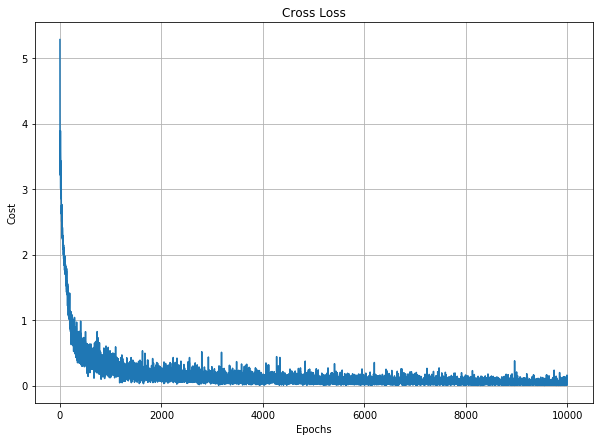训练的准确率
[外链图片转存失败,源站可能有防盗链机制,建议将图片保存下来直接上传(img-Tid5efaN-1579072744374)(output_16_2.png)]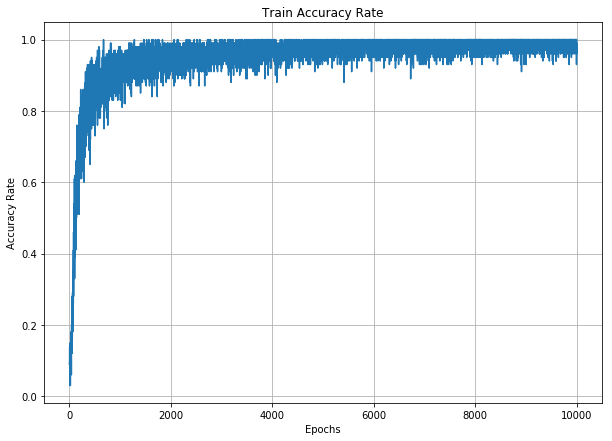训练数据中的一个样本
[外链图片转存失败,源站可能有防盗链机制,建议将图片保存下来直接上传(img-akgag6qr-1579072744380)(output_16_3.png)]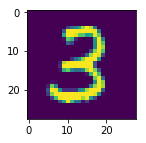第一个卷积层提取的特征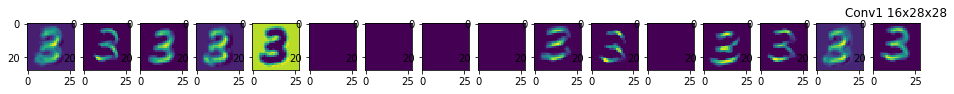2x2池化后的特征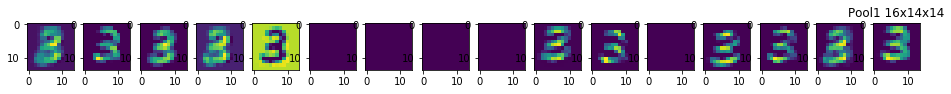第二层卷积提取特征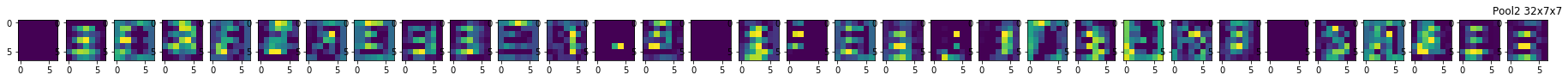2x2池化后的特征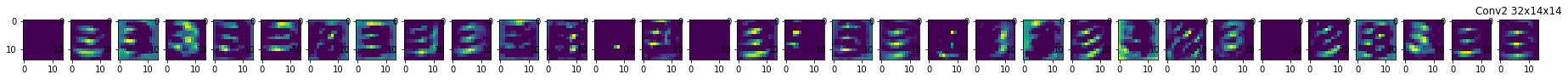安利一下，公众号展开全文• 可视化卷积神经网络的中间层时，只考虑卷积层和池化层，因为只有这两类网络层的输出是特征图；但到了全连接层，输入已经被“压平”成一个数组，不适合可视化了。 import numpy as np import keras from keras....
1、卷积神经网络中间层可视化
当可视化卷积神经网络的中间层时，只考虑卷积层和池化层，因为只有这两类网络层的输出是特征图；但到了全连接层，输入已经被“压平”成一个数组，不适合可视化了。
import numpy as np
import keras
from keras.datasets import mnist
from keras.models import load_model,Model
from keras import backend as K
from matplotlib import pyplot as plt

(x_train,y_train),(x_test,y_test)=mnist.load_data()
image=x_train
plt.imshow(image)

#把训练好的卷积神经网络模型加载出来
model=load_model('mnist_cnn.h5')
print(model.summary())
# 中间层的可视化 首先提取前3层的输出，因为这个模型只有前3层是卷积层和池化层
layer_outputs=[layer.output for layer in model.layers[:3]]
#使用Model创建一个模型，这个模型的输入和原模型一致，但有多个输出 就是所提取的前3层
activation_model=Model(inputs=model.input,outputs=layer_outputs)
#此时输入一张图像 模型将会返回原模型前3层的结果
'''
先将这张图像转换成要求的输入形状和尺寸
然后再输入到定义好的新模型中
这个模型会有3个输出 对应前3层中每一层网络的结果
first_layer_activation 第一层 （1，26，26，32）矩阵 特征图的尺寸26*26 有32个通道即32个特征图
'''
image=np.reshape(image,(1,28,28,1))
activations=activation_model.predict(image)
# first_layer_activation=activations
# plt.matshow(first_layer_activation[0,:,:,1],cmap='viridis')
layer_names=[]
for layer in model.layers[:3]:
layer_names.append(layer.name)
images_per_row=16#绘图时将16张图放在一行
for layer_name,layer_activation in zip(layer_names,activations):
#特征图的形状为 （1,size,size,n_features）
n_features=layer_activation.shape[-1]
size=layer_activation.shape
#定义一个网格 把特征图平铺在这个上面
n_cols=n_features//images_per_row
display_grid=np.zeros((size*n_cols,images_per_row*size))
for col in range(n_cols):
for row in range(images_per_row):
channel_image=layer_activation[0,:,:,col*images_per_row+row]
channel_image-=channel_image.mean()
channel_image/=channel_image.std()
channel_image*=64
channel_image+=128
channel_image=np.clip(channel_image,0,255).astype('uint8')
display_grid[col*size:(col+1)*size,row*size:(row+1)*size]=channel_image
scale=1./size
plt.figure(figsize=(scale*display_grid.shape,scale*display_grid.shape))
plt.title(layer_name)
plt.grid(False)
plt.imshow(display_grid,aspect='auto',cmap='viridis')
plt.savefig("layer_name"+layer_name+".jpg")

Model: "sequential"
_________________________________________________________________
Layer (type)                 Output Shape              Param #
=================================================================
conv2d (Conv2D)              (None, 26, 26, 32)        320
_________________________________________________________________
conv2d_1 (Conv2D)            (None, 24, 24, 64)        18496
_________________________________________________________________
max_pooling2d (MaxPooling2D) (None, 12, 12, 64)        0
_________________________________________________________________
dropout (Dropout)            (None, 12, 12, 64)        0
_________________________________________________________________
flatten (Flatten)            (None, 9216)              0
_________________________________________________________________
dense (Dense)                (None, 128)               1179776
_________________________________________________________________
dropout_1 (Dropout)          (None, 128)               0
_________________________________________________________________
dense_1 (Dense)              (None, 10)                1290
=================================================================
Total params: 1,199,882
Trainable params: 1,199,882
Non-trainable params: 0
_________________________________________________________________
None2、卷积神经网络过滤器可视化
思想：对输入图像做梯度上升。
先输入一个空白图像，并通过梯度来更新该输入图像的值，目的是使指定的过滤器的损失值最大化，这代表了过滤器对输入图像的响应最大化。经过这一梯度上升的过程，将会得到使指定过滤器具有最大响应的图像。即指定过滤器对这一类型的图像非常敏感，对于给定的输入图像，该过滤器更容易提取到图像中这方面的特征。


展开全文• 看了CS231的课程，想自己尝试做下可视化，权当加深对卷积神经网络的理解吧。 可视化第一层卷积核 课程： 课程里提到第一层卷积一般做的是传统视觉的工作，主要提取一些有向边，可视化卷积核的原因是类似模板匹配的...
• （5）卷积神经网络的可视化有哪几类 （6）如何实现卷积神经网络的可视化 把握好以上几点，我相信你一定会有感想的 2.本人现在的研究方向是：图像的语义分割，如果有志同道合的朋友，可以组队学习 haiyangpengai@...
• 卷积神经网络】之热度图可视化 一、内容 深度学习一直被人们称为**“黑盒子”**，即内部...1.卷积核输出的可视化(Visualizing intermediate convnet outputs (intermediate activations)，即可视化卷积核经过激活...
• 可视化中间激活，是指对于给定输入，展示网络中各个卷积层和池化层输出特征图 （层输出通常被称为该层激活，即激活函数输出）。 读取图片 #加载保存模型 from keras.models import load_model model = ...深度学习
• 基于反卷积实习卷积神经网络的特征可视化可视化的网络模型为VGG-19，将每一卷积层的特征图可视化，数量可以自己设置。
• 一、可视化卷积神经网络的中间输出（中间激活）：有助于理解卷积神经网络连续的层如何对输入进行变换，也有助于初步了解卷积神经网络每个过滤器的含义。 二、可视化卷积神经网络的过滤器：有助于精确理解卷积神经...
• 卷积神经网络可视化 该存储库包含许多在PyTorch中实现的卷积神经网络可视化技术。... 我提出以下对抗性例如生成技术从敌对的东西分开的可视化。 - Fast Gradient Sign, Untargeted  - Fast Gradien
• 卷积神经网络过程可视化方面论文，非常详细，香港科技大学最新研究成果
• 卷积网络可视化与可解释性相关资料一些整理，不断更新中~ 主要作用 进一步理解CNN 帮助我们分析训练好网络 学会如何生成热力图，如下图: 博客 Distill 非常推荐一个网站 Global Average ...深度学习 可解释性
• 目录标题中间特征层可视化导入ImageNet VGG16网络加载任一图片构建多特征层输出模型所有中间层显示参考 中间特征层可视化 导入ImageNet VGG16网络 导入基础包 import numpy as np import matplotlib.pyplot as plt...深度学习 tensorflow...